Courses
Courses for Kids
Free study material
Offline Centres
More

# NCERT Solutions for Class 2 Maths Chapter 7 - Jugs And MugsLast updated date: 24th Nov 2023
Total views: 480.3k
Views today: 11.80k

## Download NCERT Solutions for Class 2 Maths Chapter 7: Free PDF

NCERT Solutions for Class 2 Maths Chapter 7 - Jugs and Mugs are available on this page in Vedantu. These solutions are designed as per the latest syllabus of NCERT. This free PDF will cover all NCERT questions that are provided in the Class 2 Maths textbook in Chapter 7 with precise solutions. All the solutions are explained clearly in a simple way. Kids can refer to these solutions and even parents can help their kids by explaining these solutions to them. These NCERT Solutions for NCERT Class 2 Maths Chapter 7, can be downloaded for free in a PDF format.

## Access NCERT Solutions for Class 2 Maths Chapter 7 – Jugs and Mugs

1. Guess and Find:

(a) How many drops of lemon juice do you get from  half a lemon? _______

Ans: From half a lemon, we can get $7 - 8$ drops.

(b) How many drops of lemon juice do you get from  a full lemon? __________

Ans: We can get $15 - 16$ drops of lemon.

(c) How many drops of lemon juice fill one  spoon? ____________

Ans: It will take $12 - 13$ drops of lemon juice to fill the spoon.

2. For making $6$ glasses of lemon drink:

(a). How many lemons will you need? ____________

Ans: $2 - 3$ lemons are needed to make $6$ glasses of lemon drink

(b). How many spoons of sugar will you take? ____________

Ans: I will take  $5 - 6$ spoons of sugar .

3. Do you think it is easier to pour into a   glass from a  jug than a bucket?

Ans: Yes, pouring lemon drink to glasses from a jug is better than bucket because it is light and can be carried everywhere easily.

4. Nazim found that two big glasses fill the jug.  Shabnam wants to buy one jug full of lemon drink. How much does she need to pay? ____________

Ans: One big glass lemon juice should be Rs $10$ .

So, two big glasses of lemon juice will be $10 + 10 = Rs\,20$

Therefore, Shabnam has to pay Rs $Rs20$ for a $1$ jug ($2$ glasses) of lemon juice.

5. Chaitra found that two  small glasses fill one  big glass. How many  small glasses of lemon drink will fill the  jug? ___________

Ans: Chitra has two glasses equal to a $1$ big glass. So $2 \times 2$ small glasses of lemon drink can fill the jug. Therefore, $4$ glasses of lemon drink will fill the jug.

6. How many small glasses will fill half the jug?

Ans: If $4$ small glasses are required to fill $1$  jug then $2$ small glasses will be required to fill half jug.

Fun in Filling Vessels

7. Sunaina and Jaspreet brought different vessels from the kitchen. They had a  jug, a  glass, a  mug, a  pot and a  bowl. They filled each of these with a cup.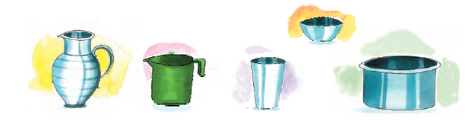Different vessels in the kitchen

(a) Guess which vessel holds the least water. ____________

Ans: From the above image, here the bowl is the smallest of all other vessels, So it  will hold the least water.

(b) Which vessel holds the most water? ___________

Ans: Here, the pot will hold the most water.

8. Now, you collect different vessels from your kitchen. Use the same cup to fill each of them. Count the number of cups of water each of them holds. Ans: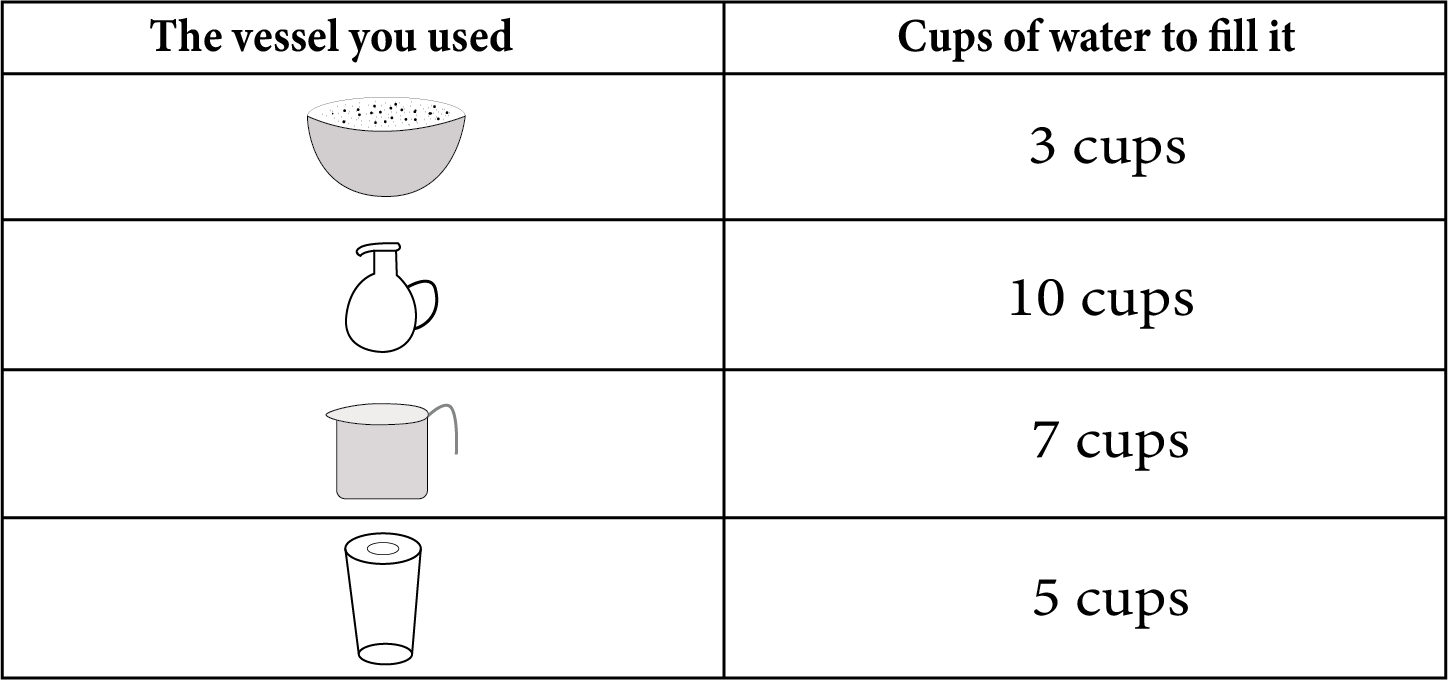Different vessels

9. Right the one which holds the most water.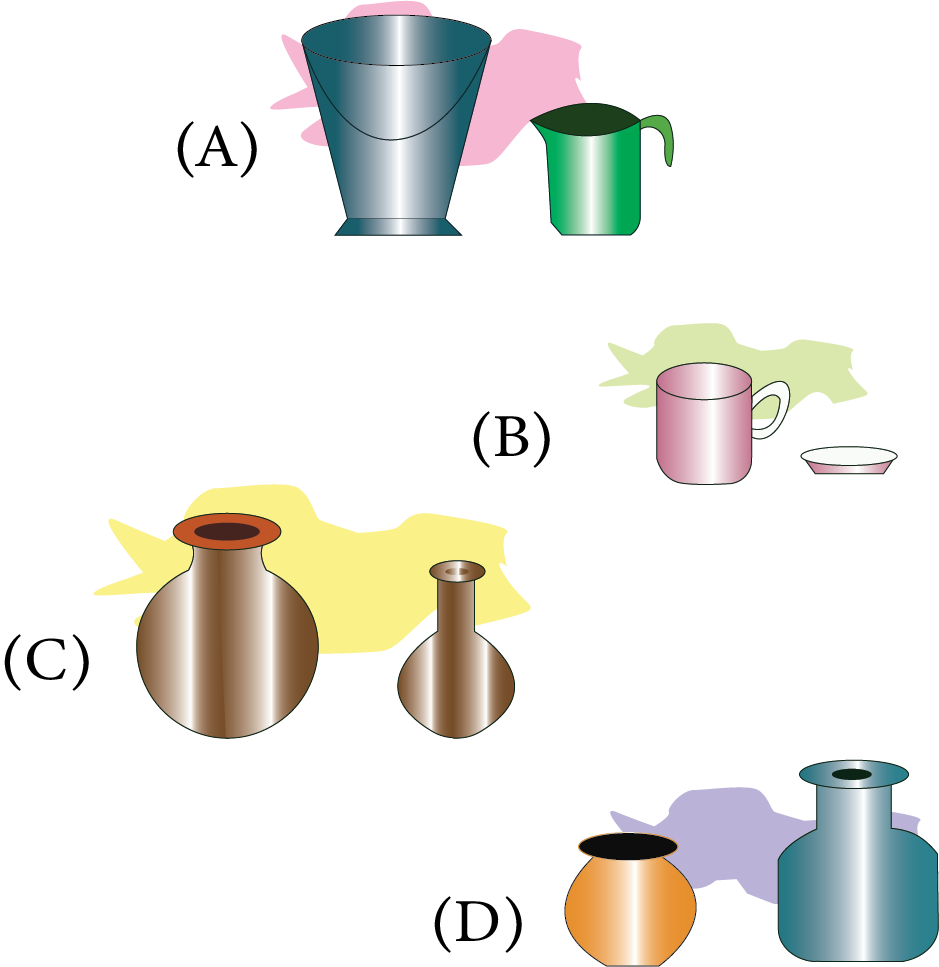Which holds the water more

Ans: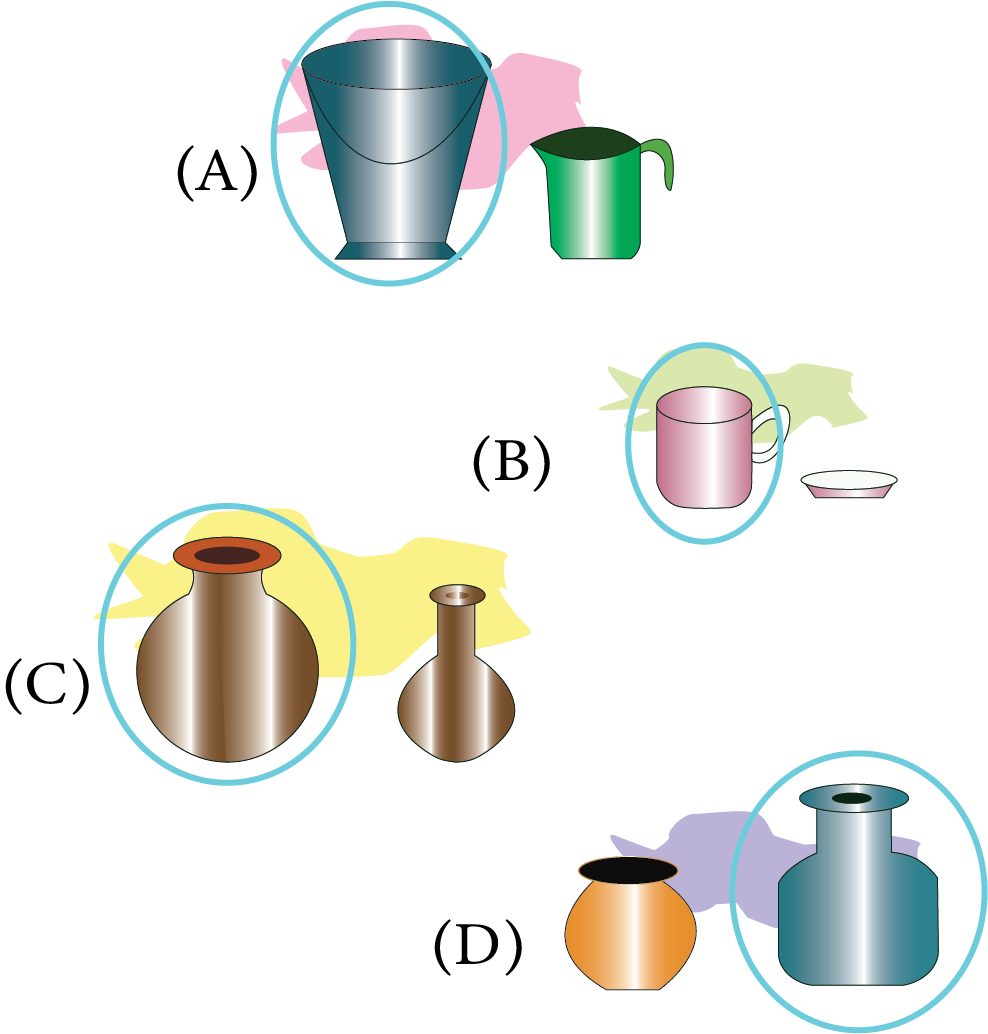Which object holds the water more

Rani and the Milkman

10. One day, Rani’s mother gave her a different pot to take milk. Every day,  Rani’s pot would fill to the top. But today, Rani found that the pot was not filled completely.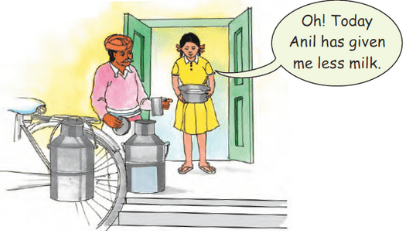Rani and the Milkman

(a) Do you think Anil has given Rani less milk? Help her.

Ans:

No, I don't think Anil has given her less milk. It seems the pot with Rani is bigger than her usual pot. Thus, the pot is not filled up to the brim.

(b) Find out how much milk does your mother buy every day? ____________

Ans: My mother buys half litre $\left( {\dfrac{1}{2}} \right)$ milk every day.

Water is Useful

11. Find out how much water (in mugs or buckets) is used in your house for each of the following. Guess and then find out: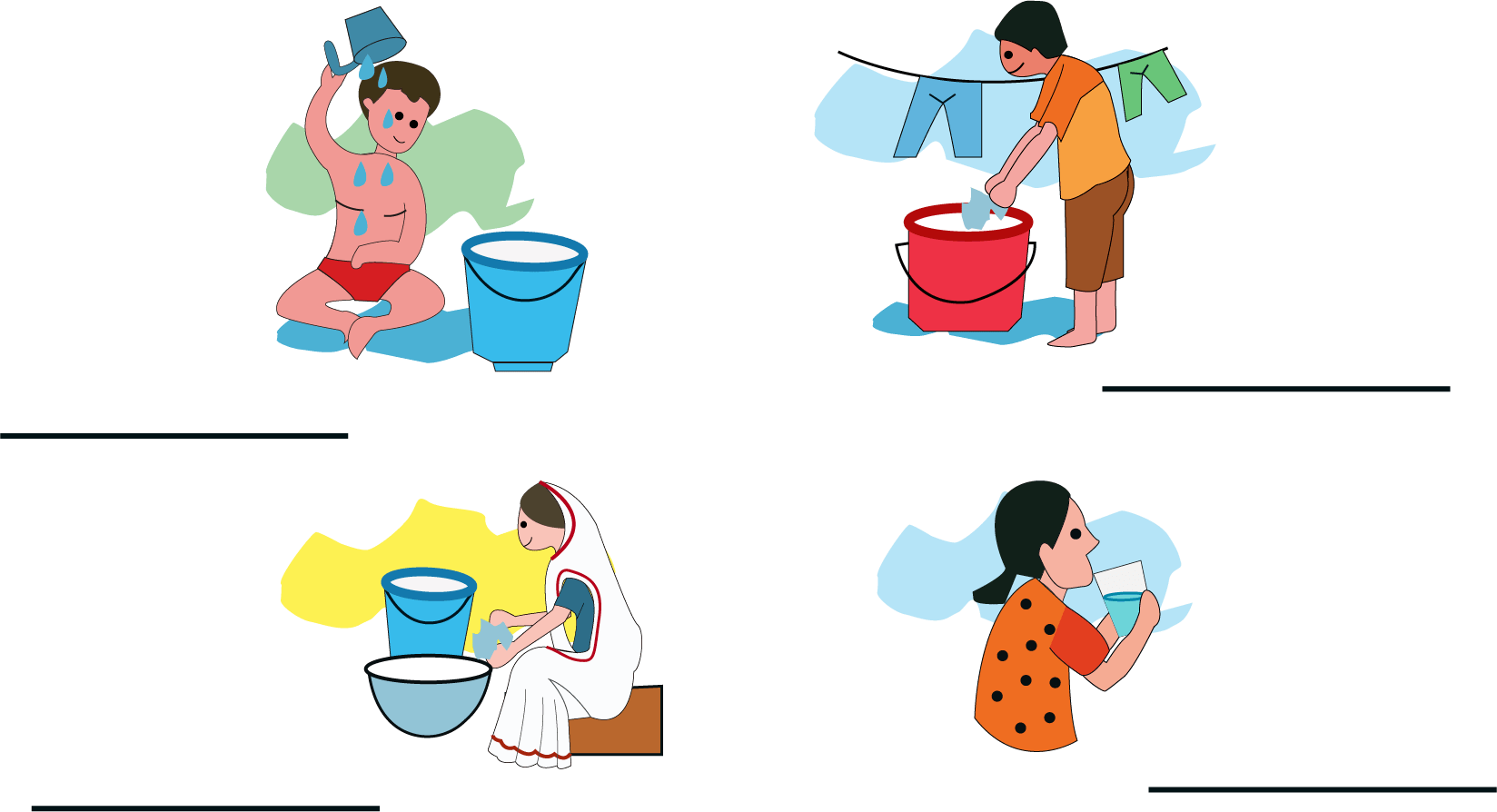Family member using water

Ans: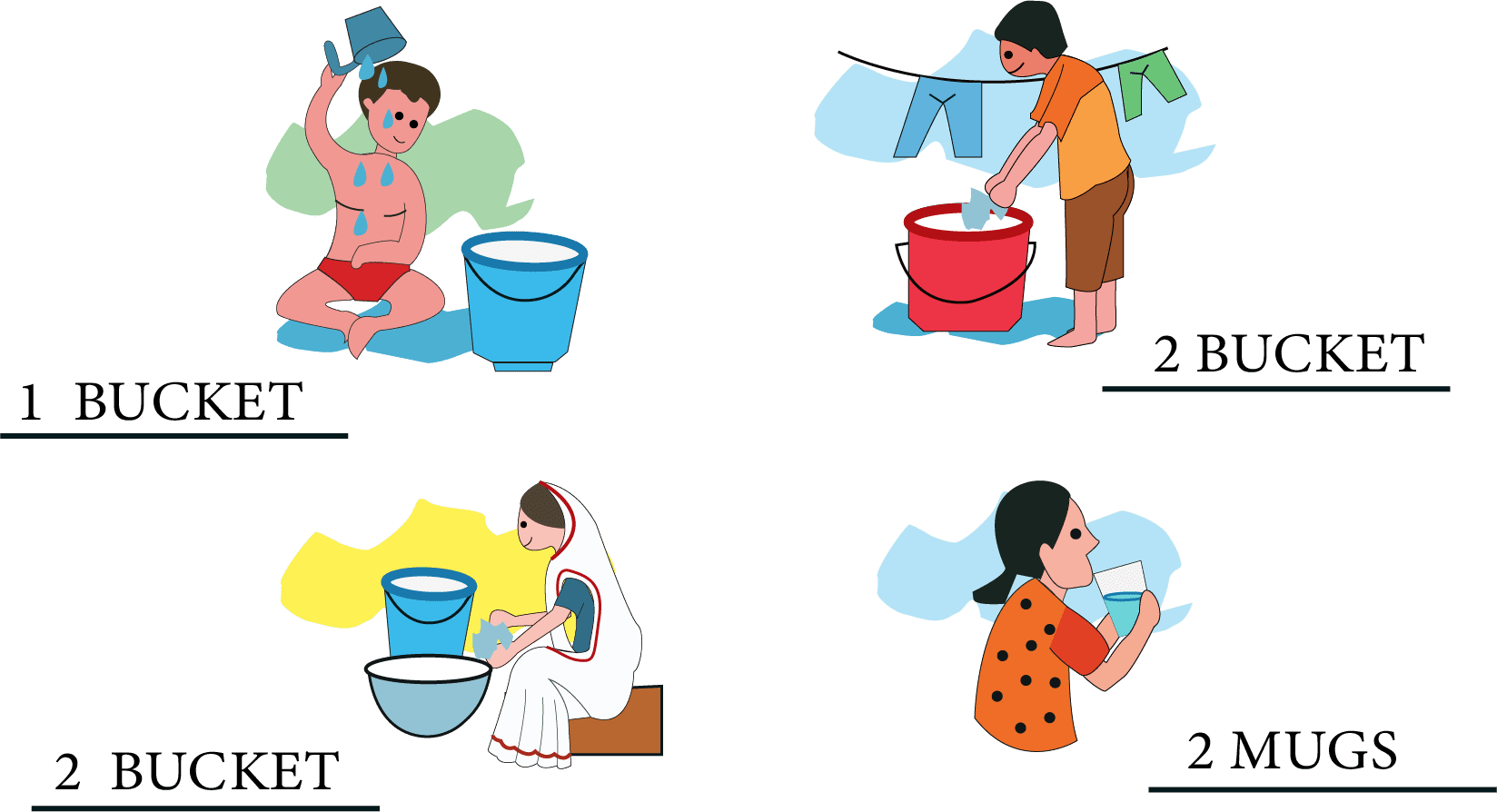Number of buckets used by family members

12. Guess and then find out:

(a) How many mugs of water fill one bucket? ______________

Ans: $20$ mugs of water can fill one bucket.

(b) How many buckets of water do you use to take a bath? ______________

Ans:  It took $1$ and a half bucket of water to take a bath.

(c) How many mugs of water do you use to take a bath? ______________

Ans: $25 - 30$ mugs of water is required to take a bath.

13.

(a) How much water can fill a balloon?

Ans: $1$ mug of water can fill a balloon.

(b) How much water is needed to fill a water gun (pichkari) ?

Ans: It will require $2$ mugs of water are needed to fill a water gun.

(c) One camel can drink $9$ buckets of water at once. How many buckets of water can two camels drink?

Ans; It is given that $1$ camel $= 9$ buckets of water. So $2$ camels $= 2 \times 9$ buckets of water. So two camels can drink $18$ buckets of water at once.

## A Glimpse of Class 2 Maths Chapter 7: Jugs and Mugs

This chapter mainly focuses on measuring and comparing the capacity of containers, used in daily life, in terms of non-standard units. NCERT Class 2 Chapter 7 Jugs and Mugs, helps students to get the basic idea of a unit of volume. The exercises in this chapter give a rough idea about how much a litre stands for. To learn using standard measuring units, such as 1 litre, 500 ml, 200 ml, etc., students can rely on Class 2 Maths Chapter 7 NCERT Solutions. The following exercises are covered under CBSE Class 2 Maths Chapter 7 Solutions.

## Important Questions and Answers of Chapter 7 Class 2 Maths

Q1. A 1 litre bottle will be filled with 5 glasses of water. How many glasses of water is required to fill a 2 litre water bottle?

Ans: With 5 glasses of water we can fill 1 litre water bottle.

2 litre = 1liter +1 litre

= 5 glasses + 5 glasses

So, to fill a 2 litre water bottle we need 10 glasses of water.

Q2. If half a lemon is needed to prepare one glass of lemon drink, how many lemons will be needed to make 4 glasses of lemon drink?

Ans: From half a lemon, we can make 1 glass of lemon drink.

With half + half i.e. 1 lemon, we can prepare 2 glasses of lemon drink.

Therefore, we will need 1 + 1 = 2 lemons to prepare 4 glasses of lemon drink.

## Key Features of NCERT Solutions for Class 2 Maths Chapter 7 - Jugs and Mugs

Our experienced teachers have a forte in preparing the NCERT Solutions For Class 2 Maths in simple language and to-the-point. The benefits of our NCERT Solutions for Class 2 Maths are as follows:

• All the solutions in this PDF are written by subject experts at Vedantu.

• The objective of this chapter is also explained clearly.

• All solutions are zero error answers with 100% reasoning.

• All solutions are explained in detail and check kids attention to detail.

• All explanations are simple and easy to understand.

• Provides in-depth knowledge with shortcuts, tips, alternative methods & points to remember.

• Acts as a quick brush up for the concepts with important definitions and formulas on the chapters.

• Even an infinitesimal doubt can be addressed by referring to our NCERT Solutions.

• NCERT Solutions for Class 2 Maths Chapter 7 - Jugs and Mugs helps in developing good basics for kids.

• These NCERT solutions are free to download in a PDF format.

## Class 2 Maths Chapter-wise Marks Weightage

The CBSE Class 2 syllabus comprises many concept building and application-based chapters that are crucial for a strong foundation in mathematics. Here, we have listed down the chapters based on their weightage in the curriculum-

 Chapter No. Chapter Marks 1 What is Long, What is Round? 4 2 Counting in Groups 10 3 How Much Can You Carry? 8 4 Counting in Tens 5 5 Patterns 10 6 Footprints 7 7 Jugs and Mugs 8 8 Tens and Ones 10 9 My Funday 5 10 Add our Points 8 11 Lines and Lines 7 12 Give and Take 5 13 The Longest Step 5 14 Birds Come, Birds Go 5 15 How many Ponytails? 3

## Conclusion

Students can access these solutions to learn the concept in an easy manner. Every explanation is in easy language and accurate as they are prepared by subject experts. Learning the concepts Class 2 Maths Chapter 7 Jugs And Mugs are important while understanding the concepts of measurement and comparing capacity in mathematics. Students learn to measure the capacity of things in this chapter, especially liquids.

They also learn the units and measurements involved with the quantity and capacity. Students can download solutions for this chapter and can easily trail into the solution as there is a detailed chronological approach towards problem-solving.

## FAQs on NCERT Solutions for Class 2 Maths Chapter 7 - Jugs And Mugs

1. What Concept is Covered in the NCERT Class 2 Maths Chapter 7?

NCERT Class 2 Maths Chapter 7 Jugs and Mugs focuses on teaching the basics of volume and measurement, using daily life scenes such as in a market, fair, and saving water. The examples of different sized containers used in the chapter teaches the significance of units in a very lucid way.

2. How Can You Get Access to NCERT Solutions For Class 2 Maths Chapter 7?

3. How Can You Excel in CBSE Class 2 Mathematics?

Regular practice is the key to success. However, referring to notes and solutions from Vedantu builds a strong base and saves revision time. You can quickly verify your answers by referring to these free solutions as and when you practice.

4. Is Chapter 7 of NCERT Class 2 Maths easy?

Ans: Chapter 7 of NCERT Class 2 Maths is easy. In fact, if you learn the main and basic concepts of a chapter, every chapter will seem really easy to you. Maths is a really interesting and scoring subject. Students fear Maths for no reason. Once the students start to understand the basic concept behind every Math topic, they will find Maths to be a very easy subject. So yes, the key to easy Maths learning is understanding.

5. How can I study Chapter 7 of NCERT Class 2 Maths?

You can study Chapter 7 of NCERT Class 2 Maths in the following ways-

• Try to understand the concept behind the chapter. Maths is a conceptual subject. Knowing the concepts will help the students grasp Maths topics very easily.

• Get all of your doubts cleared up from your subject teacher. Do not ever hesitate to ask your teacher if you face any doubts.

• Practise all the exercises given in your textbook. The more you practise, the better you will get.

6. How can I ace Chapter 7 of NCERT Class 2 Maths?

You can score good marks or even top in your Chapter 7 of NCERT Class 2 Maths exam in the following ways-